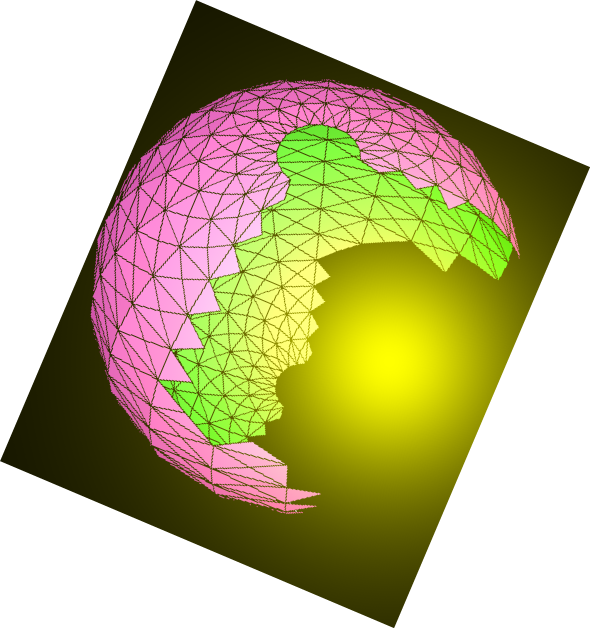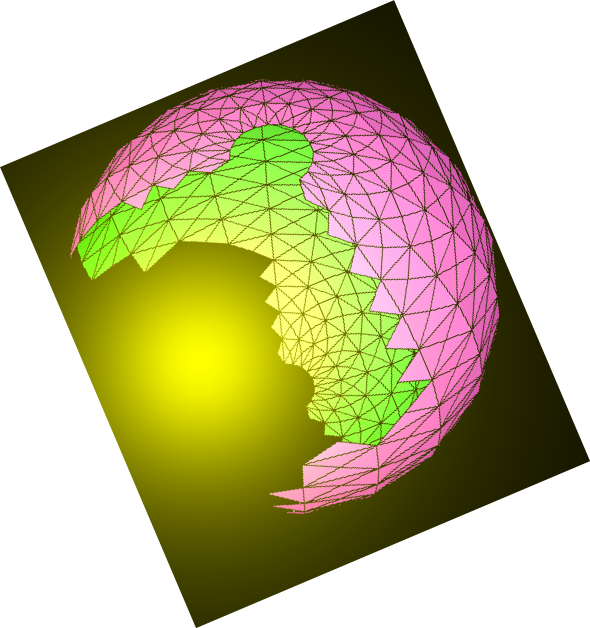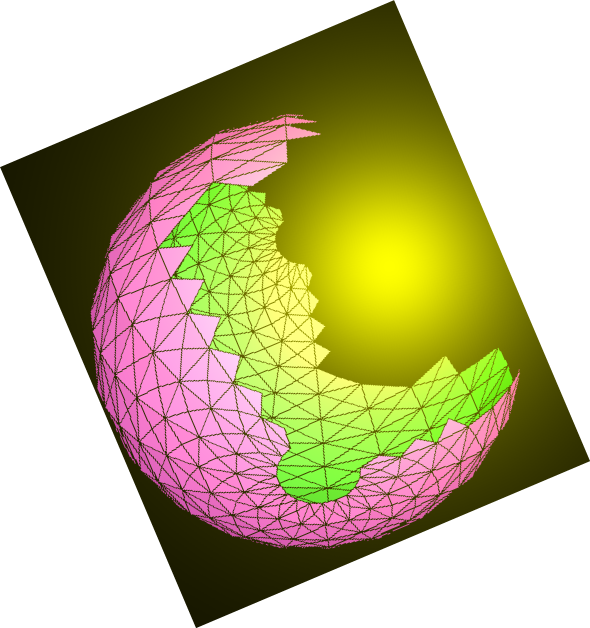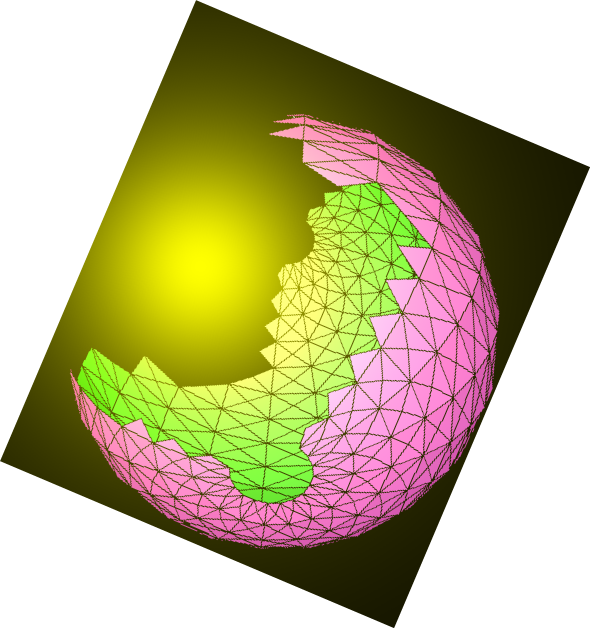# N.S.B. Cosmic Center# JavaScript & MathScript Interactive Document Generator-Demo-02

This document is generated by N.S.B. JavaScript & MathScript Interactive Document Generator version 001.

Introduction to N.S.B. JavaScript & MathScript Interactive Document Generator

JavaScript Data Types

Now that you know how to input and execute JavaScript code, let us examine some data types and some associated manipulations.

This sequence defines a number, examines and determines its type:

###### output(5)=> true

Notice that typeof() is a basic JavaScript function. On the other hand,the functions isInt() and isFlt() are defined by our program. The function isInt() checks to see whether the data type is an integer; the function isFlt() checks to see whether it is "float", which means it has a decimal point. There is another function isXpn(); this checks whether a number has exponential notation. Consider these:

###### output(6)=> 1.989e+30

Many of the physical constants (such as speedOfLight and constGravity) and related functions (such as mass()) are already defined in the program. Let us first check the numeric types of these, without seeing them.

###### output(13)=> true

Let us verify the above statements by seeing our numbers. Putting them in a JavaScript array:

###### output(14)=> [ 299792458, 6.673e-11, 1.989e+30 ]

Hence, we see that c is given as a whole integer; while G and m are given as exponential numbers.

Now that we have defined the above physical constants, let us compute the well-known Schwarzschild radius of the sun:

###### output(15)=> 2953

Notice that all our physical quantities are given in the MKS or SI system of units. Hence the above radius is given in meters. The followings give the above result in kilometers, in miles, and in centimeters:

###### output(18)=> 2.953e+5

We shall continue our exploration of JavaScript data type in the next document.

###### NextHome
N.S.B. Cosmic Center